particle accelerator

(redirected from Ring collider)
Also found in: Dictionary, Thesaurus.
Related to Ring collider: particle accelerator

particle accelerator,

apparatus used in nuclear physics to produce beams of energetic charged particles and to direct them against various targets. Such machines, popularly called atom smashers, are needed to observe objects as small as the atomic nucleusnucleus,
in physics, the extremely dense central core of an atom. The Nature of the Nucleus
Composition

Atomic nuclei are composed of two types of particles, protons and neutrons, which are collectively known as nucleons.
in studies of its structure and of the forces that hold it together. Accelerators are also needed to provide enough energy to create new particles. Besides pure research, accelerators have practical applications in medicine and industry, most notably in the production of radioisotopes. A majority of the world's particle accelerators are situated in the United States, either at major universities or national laboratories. In Europe the principal facility is at CERNCERN
or European Organization for Nuclear Research,
nuclear and particle physics research center straddling the French-Swiss border W of Geneva, Switzerland. Established in 1952 as the provisional European Center for Nuclear Research (the acronym CERN derives from this
near Geneva, Switzerland; in Russia important installations exist at Dubna and Serpukhov.

Design of Particle Accelerators

There are many types of accelerator designs, although all have certain features in common. Only charged particles (most commonly protons and electrons, and their antiparticlesantiparticle,
elementary particle corresponding to an ordinary particle such as the proton, neutron, or electron, but having the opposite electrical charge and magnetic moment.
; less often deuterons, alpha particles, and heavy ions) can be artificially accelerated; therefore, the first stage of any accelerator is an ionion,
atom or group of atoms having a net electric charge. Positive and Negative Electric Charges

A neutral atom or group of atoms becomes an ion by gaining or losing one or more electrons or protons.
source to produce the charged particles from a neutral gas. All accelerators use electric fields (steady, alternating, or induced) to speed up particles; most use magnetic fields to contain and focus the beam. Meson factories (the largest of which is at the Los Alamos, N.Mex., Scientific Laboratory), so called because of their copious pionpion
or pi meson,
lightest of the meson family of elementary particles. The existence of the pion was predicted in 1935 by Hideki Yukawa, who theorized that it was responsible for the force of the strong interactions holding the atomic nucleus together.
production by high-current proton beams, operate at conventional energies but produce much more intense beams than previous accelerators; this makes it possible to repeat early experiments much more accurately. In linear accelerators the particle path is a straight line; in other machines, of which the cyclotron is the prototype, a magnetic field is used to bend the particles in a circular or spiral path.

Linear Accelerators

The early linear accelerators used high voltage to produce high-energy particles; a large static electric charge was built up, which produced an electric field along the length of an evacuated tube, and the particles acquired energy as they moved through the electric field. The Cockcroft-Walton accelerator produced high voltage by charging a bank of capacitors in parallel and then connecting them in series, thereby adding up their separate voltages. The Van de Graaff accelerator achieved high voltage by using a continuously recharged moving belt to deliver charge to a high-voltage terminal consisting of a hollow metal sphere. Today these two electrostatic machines are used in low-energy studies of nuclear structure and in the injection of particles into larger, more powerful machines. Linear accelerators can be used to produce higher energies, but this requires increasing their length.

Linear accelerators, in which there is very little radiation loss, are the most powerful and efficient electron accelerators; the largest of these, the Stanford linear accelerator (SLAC), completed in 1957, is 2 mi (3.2 km) long and produces 20-GeV—in particle physics energies are commonly measured in millions (MeV) or billions (GeV) of electron-voltselectron-volt,
abbr. eV, unit of energy used in atomic and nuclear physics; 1 electron-volt is the energy transferred in moving a unit charge, positive or negative and equal to that charge on the electron, through a potential difference of 1 volt.
(eV)—electrons. SLAC is now used, however, not for particle physics but to produce a powerful X-ray laser. Modern linear machines differ from earlier electrostatic machines in that they use electric fields alternating at radio frequencies to accelerate the particles, instead of using high voltage. The acceleration tube has segments that are charged alternately positive and negative. When a group of particles passes through the tube, it is repelled by the segment it has left and is attracted by the segment it is approaching. Thus the final energy is attained by a series of pushes and pulls. Recently, linear accelerators have been used to accelerate heavy ions such as carbon, neon, and nitrogen.

Circular Accelerators

In order to reach high energy without the prohibitively long paths required of linear accelerators, E. O. Lawrence proposed (1932) that particles could be accelerated to high energies in a small space by making them travel in a circular or nearly circular path. In the cyclotron, which he invented, a cylindrical magnet bends the particle trajectories into a circular path whose radius depends on the mass of the particles, their velocity, and the strength of the magnetic field. The particles are accelerated within a hollow, circular, metal box that is split in half to form two sections, each in the shape of the capital letter D. A radio-frequency electric field is impressed across the gap between the D's so that every time a particle crosses the gap, the polarity of the D's is reversed and the particle gets an accelerating "kick." The key to the simplicity of the cyclotron is that the period of revolution of a particle remains the same as the radius of the path increases because of the increase in velocity. Thus, the alternating electric field stays in step with the particles as they spiral outward from the center of the cyclotron to its circumference. However, according to the theory of relativity the mass of a particle increases as its velocity approaches the speed of light; hence, very energetic, high-velocity particles will have greater mass and thus less acceleration, with the result that they will not remain in step with the field. For protons, the maximum energy attainable with an ordinary cyclotron is about 10 million electron-volts.

Two approaches exist for exceeding the relativistic limit for cyclotrons. In the synchrocyclotron, the frequency of the accelerating electric field steadily decreases to match the decreasing angular velocity of the protons. In the isochronous cyclotron, the magnet is constructed so the magnetic field is stronger near the circumference than at the center, thus compensating for the mass increase and maintaining a constant frequency of revolution. The first synchrocyclotron, built at the Univ. of California at Berkeley in 1946, reached energies high enough to create pionspion
or pi meson,
lightest of the meson family of elementary particles. The existence of the pion was predicted in 1935 by Hideki Yukawa, who theorized that it was responsible for the force of the strong interactions holding the atomic nucleus together.
, thus inaugurating the laboratory study of the mesonmeson
[Gr.,=middle (i.e., middleweight)], class of elementary particles whose masses are generally between those of the lepton class of lighter particles and those of the baryon class of heavier particles. From a technical point of view mesons are strongly interacting bosons; i.
family of elementary particleselementary particles,
the most basic physical constituents of the universe. Basic Constituents of Matter

Molecules are built up from the atom, which is the basic unit of any chemical element. The atom in turn is made from the proton, neutron, and electron.
.

Further progress in physics required energies in the GeV range, which led to the development of the synchrotron. In this device, a ring of magnets surrounds a doughnut-shaped vacuum tank. The magnetic field rises in step with the proton velocities, thus keeping them moving in a circle of nearly constant radius, instead of the widening spiral of the cyclotron. The entire center section of the magnet is eliminated, making it possible to build rings with diameters measured in miles. Particles must be injected into a synchrotron from another accelerator. The first proton synchrotron was the cosmotron at Brookhaven (N.Y.) National Laboratory, which began operation in 1952 and eventually attained an energy of 3 GeV. The 6.2-GeV synchrotron (the bevatron) at the Lawrence Berkeley National Laboratory was used to discover the antiproton (see antiparticleantiparticle,
elementary particle corresponding to an ordinary particle such as the proton, neutron, or electron, but having the opposite electrical charge and magnetic moment.
).

The 500-GeV synchrotron at the Fermi National Accelerator Laboratory at Batavia, Ill., was built to be the most powerful accelerator in the world in the early 1970s, with a ring circumference of approximately 4 mi (6 km). The machine was upgraded (1983) to accelerate protons and counterpropagating antiprotons to such enormous speeds that the ensuing impacts delivered energies of up to 2 trillion electron-volts (TeV)—hence the ring was been dubbed the Tevatron. The Tevatron was an example of a so-called colliding-beams machine, which is really a double accelerator that causes two separate beams to collide, either head-on or at a grazing angle. Because of relativistic effects, producing the same reactions with a conventional accelerator would require a single beam hitting a stationary target with much more than twice the energy of either of the colliding beams.

Plans were made to build a huge accelerator in Waxahachie, Tex. Called the Superconducting Supercollider (SSC), a ring 54 mi (87 km) in circumference lined with superconducting magnets (see superconductivitysuperconductivity,
abnormally high electrical conductivity of certain substances. The phenomenon was discovered in 1911 by Heike Kamerlingh Onnes, who found that the resistance of mercury dropped suddenly to zero at a temperature of about 4.
) was intended to produce 40 TeV particle collisions. The program was ended in 1993, however, when government funding was stopped.

In Nov., 2009, the Large Hadron Collider (LHC), a synchroton constructed by CERN, became operational, and in Mar., 2010, it accelerated protons to 3.5 TeV to produce collisions of 7 TeV, a new record. The LHC's main ring, which uses superconducting magnets, is housed in a circular tunnel some 17 mi (27 km) long on the French-Swiss border; the tunnel was originally constructed for the Large Electron Positron Collider, which operated from 1989 to 2000. The LHC was shut down in 2013–15 to make improvements designed to permit it to produce collisions involving protons that have been accelerated up to 7 TeV (and collisions of lead nuclei at lower energies), and in trials in 2015 it produced collisions of 13 TeV, a further record. A new shutdown for further improvements began in 2018. In 2012 CERN scientists announced the discovery of a new elementary particle consistent with a Higgs particle; they confirmed its discovery the following year. In addition to the Higgs particle, the LHC is being used to investigate quarks, gluons, and other particles and aspects of physics' Standard Model (see elementary particleselementary particles,
the most basic physical constituents of the universe. Basic Constituents of Matter

Molecules are built up from the atom, which is the basic unit of any chemical element. The atom in turn is made from the proton, neutron, and electron.
).

The synchrotron can be used to accelerate electrons but is inefficient. An electron moves much faster than a proton of the same energy and hence loses much more energy in synchrotron radiationsynchrotron radiation,
in physics, electromagnetic radiation emitted by high-speed electrons spiraling along the lines of force of a magnetic field (see magnetism). Depending on the electron's energy and the strength of the magnetic field, the maximum intensity will occur as
. A circular machine used to accelerate electrons is the betatron, invented by Donald Kerst in 1939. Electrons are injected into a doughnut-shaped vacuum chamber that surrounds a magnetic field. The magnetic field is steadily increased, inducing a tangential electric field that accelerates the electrons (see inductioninduction,
in electricity and magnetism, common name for three distinct phenomena. Electromagnetic induction is the production of an electromotive force (emf) in a conductor as a result of a changing magnetic field about the conductor and is the most important of the
).

Particle accelerator

An electrical device which accelerates charged atomic or subatomic particles to high energies. The particles may be charged either positively or negatively. If subatomic, the particles are usually electrons or protons and, if atomic, they are charged ions of various elements and their isotopes throughout the entire periodic table of the elements.

Accelerators that produce various subatomic particles at high intensity have many practical applications in industry and medicine as well as in basic research. Electrostatic generators, pulse transformer sets, cyclotrons, and electron linear accelerators are used to produce high levels of various kinds of radiation that in turn can be used to polymerize plastics, provide bacterial sterilization without heating, and manufacture radioisotopes which are utilized in industry and medicine for direct treatment of some illnesses as well as research. They can also be used to provide high-intensity beams of protons, neutrons, heavy ions, pi mesons, or x-rays that are used for cancer therapy and research. The x-rays used in industry are usually produced by arranging for accelerated electrons to strike a solid target. However, with the advent of electron synchrotron storage rings that produce x-rays in the form of synchrotron radiation, many new industrial applications of these x-rays have been realized, especially in the field of solid-state microchip fabrication and medical diagnostics. See Radioisotope, Synchrotron radiation

Particle accelerators fall into two general classes—electrostatic accelerators that provide a steady dc potential, and varieties of accelerators that employ various combinations of time-varying electric and magnetic fields.

Electrostatic accelerators

Electrostatic accelerators in the simplest form accelerate the charged particle either from the source of high voltage to ground potential or from ground potential to the source of high voltage. All particle accelerations are carried out inside an evacuated tube so that the accelerated particles do not collide with air molecules or atoms and may follow trajectories characterized specifically by the electric fields utilized for the acceleration. The maximum energy available from this kind of accelerator is limited by the ability of the evacuated tube to withstand some maximum high voltage.

Time-varying field accelerators

In contrast to the high-voltage-type accelerator which accelerates particles in a continuous stream through a continuously maintained increasing potential, the time-varying accelerators must necessarily accelerate particles in small discrete groups or bunches.

An accelerator that varies only in electric field and does not use any magnetic guide or turning field is customarily referred to as a linear accelerator or linac. In the simplest version of this kind of accelerator, the electrodes that are used to attract and accelerate the particles are connected to a radio-frequency (rf) power supply or oscillator so that alternate electrodes are of opposite polarity. In this way, each successive gap between adjacent electrodes is alternately accelerating and decelerating. If these acceleration gaps are appropriately spaced to accommodate the increasing velocity of the accelerated particle, the frequency can be adjusted so that the particle bunches are always experiencing an accelerating electric field as they cross each successive gap. In this way, modest voltages can be used to accelerate bunches of particles indefinitely, limited only by the physical length of the accelerator construction.

All conventional (but not superconducting) research linacs usually are operated in a pulsed mode because of the extremely high rf power necessary for their operation. The pulsed operation can then be adjusted so that the duty cycle or amount of time actually on at full power averages to a value that is reasonable in cost and practical for cooling. This necessarily limited duty cycle in turn limits the kinds of research that are possible with linacs; however, they are extremely useful (and universally used) as pulsed high-current injectors for all electron and proton synchrotron ring accelerators. Superconducting linear accelerators have been constructed that are used to accelerate electrons and also to boost the energy of heavy ions injected from electrostatic machines. These linacs can easily operate in the continuous-wave (cw) rather than pulsed mode, because the rf power losses are only a few watts.

The Continuous Electron Beam Accelerator Facility (CEBAF) uses two 400-MeV superconducting linacs to repeatedly accelerate electrons around a racetrack-like arrangement where the two linacs are on the opposite straight sides of the racetrack and the circular ends are a series of recirculation bending magnets, a different set for each of five passes through the two linacs in succession. The continuous electron beam then receives a 400-MeV acceleration on each straight side or 0.8 GeV per turn, and is accelerated to a final energy of 4 GeV in five turns and extracted for use in experiments. The superconducting linacs allow for continuous acceleration and hence a continuous beam rather than a pulsed beam. This makes possible many fundamental nuclear and quark structure measurements that are impossible with the pulsed electron beams from conventional electron linacs. See Superconducting devices

As accelerators are carried to higher energy, a linac eventually reaches some practical construction limit because of length. This problem of extreme length can be circumvented conveniently by accelerating the particles in a circular path maintained by either static or time-varying magnetic fields. Accelerators utilizing steady magnetic fields as guide paths are usually referred to as cyclotrons or synchrocyclotrons, and are arranged to provide a steady magnetic field over relatively large areas that allow the particles to travel in an increasing spiral orbit of gradually increasing size as they increase in energy.

Practical limitations of magnet construction and cost have kept the size of circular proton accelerators with static magnetic fields to the vicinity of 100 to 1000 MeV. For even higher energies, up to 400 GeV per nucleon in the largest conventional (not superconducting) proton synchrotron in operation, it is necessary to vary the magnetic field as well as the electric field in time. In this way the magnetic field can be of a minimal practical size, which is still quite extensive for a 980-GeV accelerator (6500 ft or 2000 m in diameter). This circular magnetic containment region, or “racetrack,” is injected with relatively low-energy particles that can coast around the magnetic ring when it is at minimum field strength. The magnetic field is then gradually increased to stay in step with the higher magnetic rigidity of the particles as they are gradually accelerated with a time-varying electric field.

Superconducting magnets

The study of the fundamental structure of nature and all associated basic research require an ever increasing energy in order to allow finer and finer measurements on the basic structure of matter. Since the voltage-varying and magnetic-field-varying accelerators also have limits to their maximum size in terms of cost and practical construction problems, the only way to increase particle energies even further is to provide higher-varying magnetic fields through superconducting magnet technology, which can extend electromagnetic capability by a factor of 4 to 5. Large superconducting cyclotrons and superconducting synchrotrons are in operation. See Magnet

Storage rings

Beyond the limit just described, the only other possibility is to accelerate particles in opposite directions and arrange for them to collide at certain selected intersection regions around the accelerator. The main technical problem is to provide adequate numbers of particles in the two colliding beams so that the probability of a collision is moderately high. Such storage ring facilities are in operation for both electrons and protons. Besides storing the particles in circular orbits, the rings can operate initially as synchrotrons and accelerate lower-energy injected particles to much higher energies and then store them for interaction studies at the beam interaction points.

Large proton synchrotrons have been used as storage-ring colliders by accelerating and storing protons in one direction around the ring while accelerating and storing antiprotons (negative charge) in the opposite direction. The proton and antiproton beams are carefully programmed to be in different orbits as they circulate in opposite directions and to collide only when their orbits cross at selected points around the ring where experiments are located. The antiprotons are produced by high-energy proton collisions with a target, collected, stored, cooled, and eventually injected back into the synchrotron as an antiproton beam.

Electron-positron synchrotron accelerator storage rings have been in operation for many years in the basic study of particle physics, with energies ranging from 2 GeV + 2 GeV to 104 GeV + 104 GeV. The by-product synchrotron radiation from many of these machines is used in numerous applications. However, the synchrotron radiation loss forces the machine design to larger and larger diameters, characterized by the Large Electron Positron Storage Ring (LEP) at CERN, near Geneva, Switzerland (closed down in 2000), which was 17 mi (27 km) in circumference. Conventional rf cavities enable electron-positron acceleration only up to 50–70 GeV (limited by synchrotron radiation loss) while higher energies of 100–150 GeV require superconducting cavities. See Synchrotron radiation

Although circular machines with varying magnetic fields have been developed because linacs of comparable performance would be too long (many miles), developments in linac design and utilization of powerful laser properties may result in a return to linacs that will outperform present ring machines at much lower cost. As a first example, the 20-GeV electron linac at Stanford University, Palo Alto, California, has been modified to provide simultaneous acceleration of positrons and electrons to energies as high as 50 GeV, while operating in what is called the SLED mode. After acceleration the electrons and positrons are separated by a magnet, and the two beams are magnetically directed around the opposite sides of a circle so that they collide at one intersection point approximately along a diameter extending from the end of the linac across the circle. This collider arrangement is much less expensive than the 17-mi (27-km) ring at CERN and provides electron-positron collisions of comparable energies but at lower intensities.

Accelerator, Particle

a device that accelerates charged particles to high energies. The particles may be electrons, protons, atomic nuclei, or ions.

The acceleration is accomplished with an electric field, which is capable of altering the energy of particles that carry an electric charge. Since a magnetic field can change the direction of the motion of charged particles but not their speed, it is used in accelerators to control the shape of the particle trajectory. The accelerating electric field is usually generated by external devices. In what is called collective acceleration, however, charged particles are accelerated by means of fields produced by other charged particles (see). Particle accelerators should be distinguished from plasma accelerators, which produce charged-particle fluxes that, on the average, are electrically neutral.

Particle accelerators are one of the basic tools of modern physics. They are used to provide beams of primary accelerated charged particles and beams of the secondary particles—such as mesons, neutrons, and photons—that are produced when primary accelerated particles interact with matter. High-energy particle beams are employed in the study of the nature and properties of elementary particles and find application in nuclear and solid state physics. They are also coming into increasing use in research in such fields as chemistry, biophysics, and geophysics. The importance of particle accelerators in various energy ranges is growing in other areas as well. In metallurgy, for example, accelerators are used to detect flaws in parts and structures. The wood-products industry employs accelerators to carry out rapid high-quality working of articles. In the food-processing industry accelerators are used to sterilize food products. In medicine accelerators find application in radiotherapy and “bloodless surgery.”

The development of particle accelerators was stimulated by the need for fluxes of high-energy charged particles to carry out investigations of the structure of the atomic nucleus. Natural charged-particle sources, that is, radioactive elements, were originally used, but they were limited both in the intensity and the energy of the particles emitted. In 1919, E. Rutherford used a flux of alpha particles from a radioactive source to produce the first artificial transmutation of nuclei. This achievement gave rise to a search for methods of producing beams of accelerated particles.

In the initial period of accelerator development, which lasted from 1919 to 1932, efforts were concentrated on the obtaining of high voltages and the use of such voltages to carry out the direct acceleration of charged particles. The American physicist R. Van de Graaff built an electrostatic generator in 1931, and the British physicists J. Cockcroft and E. Walton of Rutherford’s laboratory developed a type of cascade generator in 1932. These devices made it possible to produce fluxes of accelerated particles with an energy of the order of 1 million electron volts (MeV). A nuclear reaction induced by artifically accelerated particles—the splitting of the lithium nucleus by protons—was accomplished for the first time in 1932.

The years from 1931 to 1944 were a period of the inception and development of the resonance method of acceleration. In this method the particles being accelerated pass many times through an accelerating gap; they thus can acquire a high energy even when a moderate accelerating voltage is used. The cyclotron, a circular resonance accelerator invented by E. O. Lawrence, soon surpassed electrostatic accelerators in particle energy. By the end of the period a proton energy of the order of 10–20 MeV had been achieved in cyclotrons. Although resonance acceleration is also possible in linear accelerators, linear resonance accelerators did not come into use in this period because of the inadequate development of radio engineering. In 1940 the American physicist D. W. Kerst built a circular induction accelerator, called the betatron, for electrons; the idea of such an accelerator had been advanced by the American physicist J. Slepian in 1922 and the Swiss physicist R. Wideröe in 1928.

The development of phase-stable, or synchronous, accelerators began in 1944, when the Soviet physicist V. I. Veksler discovered the principle of phase stability; the principle was independently discovered slightly later by the American physicist E. M. McMillan. The application of the phase stability principle to resonance accelerators makes possible a considerable increase in the energy of the accelerated particles. Such new types of resonance accelerators as the electron synchrotron, synchrocyclotron, proton synchrotron, and microtron were proposed on the basis of the principle. At the same time, advances in radio engineering made possible the building of efficient linear resonance accelerators of electrons and heavy charged particles.

The principle of alternating-gradient focusing of particles was proposed by the Greek engineer N. Christofilos in 1950 and the American scientists E. Courant, M. Livingston, and H. Snyder in 1952. Alternating-gradient focusing permitted a substantial increase in the upper limit of the energies attainable in circular and linear accelerators. In 1956, Veksler published a paper in which he advanced the notion of the coherent, or collective, method of particle acceleration.

The following two decades saw the realization of the ideas mentioned above and the achievement of technical improvements in particle accelerators. Linear resonance accelerators proved to be the most promising machines for electron acceleration. The largest such accelerator went into operation at Stanford University in the USA in 1966. Constructed under the direction of the American physicist W. Panofsky, it is capable of accelerating electrons to an energy of 22 gigaelectron volts (GeV). The highest proton energies have been achieved in proton synchrotrons. In 1957 a 10-GeV proton synchrotron, which at the time was the largest in the world, went into operation at Dubna in the USSR. A few years later, alternating-gradient proton synchrotrons with energies of 25–30 GeV were completed in Switzerland and the USA. In 1967 a 76-GeV proton synchrotron went into operation near Serpukhov in the USSR; it was for several years the largest in the world. A 200-GeV proton synchrotron was completed in the USA in 1972; design improvements enabled it to reach 400 GeV. Work on accelerators for the energy range from 1,000 to 5,000 GeV is at various stages of design or construction in the USSR and the USA.

The lines of development of particle accelerators at the present time include increasing the energy of the accelerated particles, increasing the intensity (current) and pulse duration of the accelerated beam, and improving beam quality (reducing the spread in energy, transverse coordinates, and velocities). Along with the development of new acceleration methods, improvements are being made in traditional methods. For example, work is being carried out on the use of superconducting materials and associated low-temperature equipment in magnets and accelerating systems in order to reduce sharply power consumption and the dimensions of magnet systems. The range of application of automatic control methods in accelerators is being expanded. Accelerators are being supplemented with storage rings that permit the investigation of elementary interactions in colliding beams (see). In improving traditional acceleration methods, particular attention is paid to reducing the cost of the accelerators.

Particle accelerators may be classified in different ways. With respect, for example, to the type of particles being accelerated, electron accelerators, proton accelerators, and ion accelerators are distinguished.

With respect to the character of the particle trajectories, a distinction is made between linear accelerators and circular, or cyclic, accelerators. In linear accelerators, which are also known as linacs, the particle trajectories are close to a straight line; in circular accelerators the particle trajectories are close to a circle or spiral.

Depending on the character of the accelerating field, particle accelerators are divided into resonance and nonresonance accelerators. In resonance machines acceleration is accomplished with an alternating radio-frequency electromagnetic field; for successful acceleration to occur, the particles must move in resonance with the field. In nonresonance accelerators the direction of the field does not change during acceleration. Nonresonance machines are divided into induction accelerators and high-voltage accelerators. In induction accelerators the accelerating electric field is generated through a change in the magnetic field: the particles are accelerated by the induced electromotive force. In high-voltage accelerators the accelerating field is due to a directly applied potential difference.

Depending on the focusing mechanism, which ensures stability of particle motion in directions perpendicular to the orbit, a distinction is made between constant-gradient, or weak-focusing,

Table 1. Classification of circular accelerators (accelerators with circular or spiral-like particle trajectories)
Character of accelerating fieldMagnetic guide fieldFrequency of accelerating fieldFocusingType of acceleratorParticles accelerated
Table 2. Classification of linear accelerators (accelerators with straight-line particle trajectories)
Character of accelerating fieldFrequency of accelerating fieldType of acceleratorParticles accelerated
Nonresonance, electrostaticElectrostatic accelerator, cascade acceleratorProtons, electrons
Nonresonance, inductionLinear induction acceleratorElectrons
ResonanceConstantLinear resonance acceleratorProtons, electrons

accelerators, in which the focusing force is constant along the trajectory (at least with respect to sign), and alternating-gradient, or strong-focusing, accelerators, in which the focusing force changes sign along the trajectory—that is, focusing and defocusing sections alternate.

Circular resonance accelerators may be further classified according to the character of the controlling magnetic field (the guide field) and the accelerating electric field. The magnetic field may be constant or vary with time, and the frequency of the accelerating field may be constant or vary with time.

The classification of particle accelerators is summarized in Tables 1 and 2. Two types of machines are not encompassed by the given classification: colliding-beam machines and accelerators that make use of collective acceleration methods. Colliding-beam machines represent a distinctive modification of the accelerators listed in Tables 1 and 2: particle beams from an accelerator of a given type are directed against each other so as to collide head on. Collective-acceleration machines differ from all the described accelerators with respect to the source of the accelerating field.

In a resonance accelerator a particle is continually accelerated, since it always crosses the accelerating gap at an accelerating phase of the field—that is, when the electric field is directed with the particle motion. An ideal, or equilibrium, particle always crosses the gap at the same, equilibrium phase.

In a circular accelerator the relation between a particle’s period T of revolution and the average radius <R> of the particle’s orbit is given by the equation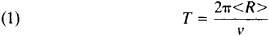where v is the speed of the particle. For the average orbit radius we have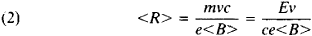Here, E = mc2 is the total relativistic energy of a particle of mass m (this energy is equal to the sum of the particle’s rest energy E0= m0c2 and its kinetic energy W, where m0 is the particle’s rest mass and c is the speed of light), e is the charge of the particle, and <B> is the average value of the magnetic induction. Consequently, the relation between the period of revolution and the particle’s energy is given by the equation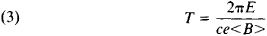For an equilibrium particle the period of revolution is equal to or a multiple of the period Ta of the accelerating field.

To fixed values of the revolution period and magnetic induction there correspond a definite equilibrium energy of the particle and equilibrium radius of its orbit. An equilibrium particle acquires in one revolution the energy eV0 cos φ0, where φ0 is the equilibrium phase (that is, the phase of the field acting on an equilibrium particle as reckoned from the field maximum) and V0 is the voltage amplitude at the gap of the accelerating electrodes. To acquire a final kinetic energy Wmax, a particle must complete N = Wmax/eV0 cos φ0 revolutions.

The distance traversed by a particle in a circular accelerator can reach tens or hundreds of thousands of kilometers. With such great path lengths, successful operation of the accelerator requires stability of equilibrium motion: small deviations of the particle with respect to phase or energy, small radial or vertical excursions of the particle, and small initial speeds in directions perpendicular to the orbit must not lead to significant departure of the particle from the equilibrium orbit. In other words, the particle must undergo an oscillatory motion about the equilibrium particle. The stability of particle motion in directions perpendicular to the orbit (the radial and vertical directions) is provided by focusing. Stability in the direction of the orbit is provided by the phase stability mechanism (phase focusing).

In a proton linear accelerator with accelerating gaps the time required for an equilibrium particle to travel between adjacent accelerating gaps is T = L/v, where L is the distance between the centers of the gaps and v is the particle speed. T is a multiple of the period of the accelerating field Ta = λ/c, where λ is the wavelength of the electromagnetic field. An energy Wmax is acquired upon passage through N = Wmax/eV0 cos φ0 accelerating gaps; this fact determines the required length of the accelerator. Since the lengths of present-day proton linear accelerators reach hundreds of meters, the problem of stability of particle motion arises; to deal with this problem, focusing and phase stability are made use of.

In order that scattering by the nuclei of gas atoms not cause particles to make substantial deviations from the equilibrium trajectory and to drop out of the acceleration process, the region around the equilibrium trajectory is enclosed by a vacuum chamber in which special pumps are used to produce a sufficiently high vacuum.

Phase focusing. Phase focusing in resonance accelerators is provided by the phase stability principle, which is associated with the energy dependence of the time interval between successive accelerations. In weak-focusing circular accelerators the period of revolution increases with increasing energy, since in equation (1) the average orbit radius increases more rapidly with increasing energy than does the particle speed. In alternating-gradient accelerators the energy dependence of the average orbit radius is much weaker. Consequently, at low energies the period of revolution usually decreases with an increase in energy (v increases faster than <R>), and at high energies the revolution period increases with increasing energy (<R> increases more rapidly than v, which is limited by the speed of light). For a period increasing with energy, the right-hand phase in Figure 1 is stable: if a particle reaches the accelerating gap at a phase φ1, > φ0, the particle will acquire an energy less than the equilibrium energy. Consequently, the period of revolution will become less than the equilibrium period, and the particle’s phase will shift so as to approach the equilibrium phase φ0. If, however, the period decreases with increasing energy, the phase φ0 is unstable, and the phase – φ0 symmetric to it is stable. In any case, if eV0 is sufficiently great, there always exists a stable equilibrium phase and a region of phases close to it within which the particles are trapped and oscillate about the equilibrium phase.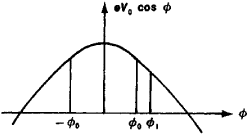Figure 1. Principle of phase stability

The increase in the energy of the equilibrium particle eV0 cos φ0 is determined by the resonance condition T = qTa, where q is an integer. For a circular accelerator the energy of the equilibrium particle is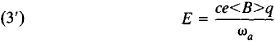where ωa = 2π/Ta is the accelerating-field frequency. Thus, the equilibrium energy can be increased by increasing the magnetic field (as in an electron synchrotron), by reducing the accelerating-field frequency (as in a synchrocyclotron), by changing both the magnetic field and the accelerating-field frequency (as in a proton synchrotron), or by varying q (as in a microtron). The law governing the variation of the magnetic field, frequency, and q determines the value of the phase φ0 for an equilibrium particle; because of phase stability, an equilibrium particle acquires precisely the energy given by equation (3’). The radius of the equilibrium orbit, which is given by equation (2), varies with energy.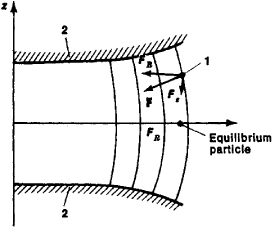Figure 2. In the presence of a “barrel-shaped” magnetic field, the magnetic force acting on a particle displaced from the equilibrium orbit has a vertically focusing component: (F) magnetic force acting on particle, (Fx) vertical component of F, (FR) radial component of F, (1) particle displaced from equilibrium orbit, (2) pole faces

For particles with a phase sufficiently close to that of an equilibrium particle, the increase in energy occurs nonuniformly, but, on the average, they acquire the same energy as the equilibrium particle. These particles are trapped in the acceleration regime. Particles that differ markedly from the equilibrium particle in phase or energy do not, on the average, acquire energy, since they are subjected sometimes to the accelerating field and sometimes to the decelerating field.

A similar mechanism of phase focusing operates in linear resonance accelerators. In this case, however, the time required to traverse the distances between adjacent gaps always decreases with increasing energy. The stable equilibrium phase thus is always – φ0.

Focusing. In circular accelerators focusing is achieved principally by special selection of the shape of the magnetic field. If the magnetic field were strictly uniform, then any deviation of particle velocity from the orbital plane would cause the particle being accelerated to depart from the equilibrium orbit in the direction of the axis of the magnet—that is, in the vertical, or z, direction. If, however, the magnetic field decreases with increasing radius, then it has a “barrel-like” shape, since in the absence of currents the magnetic field is irrotational; the force F acting on a particle consequently has a component Fz directed toward the plane of the equilibrium orbit (Figure 2).

The radial variation of the field commonly is characterized by the field index n = – ∂(ln B)/∂(ln R). Thus, for stability of motion in the vertical, or axial, direction the condition n > 0 must be satisfied—that is, the field must decrease with increasing radius. Motion in the radial direction is determined by the relation between the magnetic force eBv/c acting on the particle and the centripetal force mv2/R required to hold the particle in its orbit. In an equilibrium orbit these two quantities are equal. Should a particle with the same speed be displaced to a larger radius, to ensure radial stability the magnetic force eBvlc acting at this radius would have to be greater than mv2/R—that is, the magnetic field would have to decrease more slowly than 1/R. The same conclusion is obtained if a random displacement of the particle to a shorter radius is considered. The condition for radial stability thus imposes a limitation on the rate of decrease of the magnetic field: the field index n must be less than 1. For simultaneous stability in the radial and vertical directions, the following condition must be satisfied:

(4) 0 < n < 1

It can be shown that the radial and vertical focusing forces when this condition is fulfilled are

(5) FR = –(1 – n)mω2ΔR

Fz = – nmω2Δz

where m is the mass of the particle, ω is the angular frequency of the particle, and ΔR and Δz are the radial and vertical displacements of the particle from the equilibrium orbit. When these focusing forces operate, the particles undergo oscillations, called betatron oscillations, about the equilibrium orbit at frequencies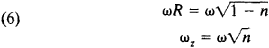These frequencies are less than ω—that is, in one orbit a particle completes less than one betatron oscillation. The focusing forces are limited by the maximum permissible values of n. Such focusing is called constant-gradient, or weak, focusing.

To increase the vertical focusing force, a strongly decreasing field must be applied (n >> 1). By contrast, to obtain a large radial focusing force, a field with large negative values of n (that is, a field that increases strongly along the radius) must be applied. These requirements cannot be fulfilled simultaneously. Under certain restrictions, however, they can be fulfilled alternately; in this way strong radial and vertical focusing can be provided. The principle of alternating-gradient focusing is based on this fact (Figure 3).

In alternating-gradient focusing the entire length of the equilibrium orbit is divided into a large number of equal sections in which magnets that provide alternate radial and vertical strong focusing are mounted. Given a certain relation among the field indexes, magnet length, and number of sections, such a system exhibits strong focusing in both transverse directions. This result can be explained physically by noting that a particle is farther from the equilibrium position in a focusing magnet than in a defocusing magnet, since the preceding defocusing magnet deflected the particle from the orbit. The action of the focusing magnets is therefore stronger than that of the defocusing magnets.

The frequency of particle oscillations under such focusing is considerably higher than the frequency of revolution, so that a particle undergoes several oscillations in a single orbit. Since an increase in focusing force leads to a decrease in the amplitude of the particle oscillations due to various driving factors, the transverse dimensions of the vacuum chamber and magnets can be decreased, and, consequently, the weight and cost of the machine can be reduced. Alternating-gradient, or strong, focusing is therefore used in all large high-energy circular accelerators.Figure 3. Arrangement of magnets in an alternating-gradient accelerator: (D) magnets that defocus in the radial direction (n >> 1), (F) magnets that focus in the radial direction (n << –1). The broken line indicates the equilibrium orbit, and the solid line indicates the orbit of a particle displaced from the equilibrium orbit.

A drawback of strong focusing is the presence of numerous resonances due to the high frequency of the particle oscillations: if the number of vertical or radial oscillations of a particle in one complete orbit or the sum or difference thereof is an integer or half-integer, resonant buildup of the oscillations occurs. For this reason high demands must be placed on the precision of magnet fabrication.

Alternating-gradient focusing is also used in linear accelerators. In this case, however, the magnetic field in the equilibrium orbit, which is a straight line, is zero. The focusing system is a set of magnetic quadrupole lenses. The magnetic field they produce is zero at the axis O of the system and increases linearly with displacement from the axis (Figure 4). The magnets focus particles in one plane (the force F is directed toward the axis) and defocus in the other (F is directed away from the axis). The focusing planes alternate from magnet to magnet, so that alternating-gradient focusing results.Figure 4. Field of a magnetic quadrupole lens: (N) north poles of magnet, (S) south poles of magnet, (F) force exerted by magnetic field on a particle moving in a direction perpendicular to the plane of the figure. At the center O, F = 0.

At low particle energies, electric field focusing is employed in addition to magnetic focusing in both circular and linear accelerators. For this purpose the accelerating electric field of the machine is made use of. The focusing principle is illustrated in Figure 5. In a conventional accelerating gap the electric field usually “sags” inward at the center of the gap. In the first half of the gap the field therefore has a focusing component directed toward the axis of the gap, and in the second half it has a defocusing component directed away from the axis of the gap. A focusing effect results if the focusing action is greater than the defocusing action. Since a particle is accelerated when it crosses the gap, it moves more rapidly, and spends less time, in the second half of the gap than in the first half. Consequently, the focusing action predominates. This effect, which is based on the change in the particle’s speed, is sometimes called electrostatic focusing. It has an appreciable magnitude only for low particle speeds, and its use in accelerators therefore is limited. The difference between the action of the electric field in the first and second halves of the gap may also be due to the time variation of the electric field (electrodynamic focusing): if the electric field decreases as the particle passes through it, the defocusing action is less than the focusing action. Focusing of this type occurs in the cyclotron and synchrocyclotron as an additional factor to magnetic focusing. In linear proton accelerators, however, the negative phase φ0 (see above) is stable. At this phase the field increases with time. The electric field in linear accelerators therefore defocuses, and special additional measures are required for particle focusing.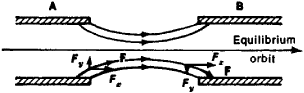Figure 5. Distribution of the electric field in the accelerating gap between two electrodes: (A) and (B) electrodes, (F) force acting on particle, (Fx) longitudinal component of F, (Fy) transverse component of F

The principle of alternating-gradient focusing can be applied to an electric field. For example, by using electrodes of complex shape it is possible to effect a change in the sign of the focusing force from gap to gap. Moreover, by also changing the sign of the equilibrium phase from gap to gap, it is possible to obtain a system with alternating-gradient focusing and alternating-sign phase stability. Such systems have been proposed and developed, but they have found extremely limited application.

When a beam being accelerated is of high intensity, the interaction between individual particles in the beam becomes significant. The repulsion of particles of like charge in accordance with Coulomb’s law leads to the weakening of the focusing forces. In circular accelerators instability of motion may also result from the synchrotron radiation emitted by the particles (see below). The interaction of charged particles has different effects in different accelerators, but it almost always determines the maximum attainable intensity; the power needed to accelerate the beam also sometimes plays a determining role.

Circular accelerators, PROTON SYNCHROTRON. The proton synchrotron is a circular resonance accelerator of protons. It has a time-varying magnetic field B and an accelerating electric field of varying frequency ωa; ωa and B vary in strict synchronism with each other so that the radius of the equilibrium orbit R remains constant. For a proton synchrotron the relation between the frequency of revolution of the particles ω = ωa/q and the average value of the magnetic induction <B> at the orbit is given by the equationThis condition follows from equations (3) and (2). It can be seen from equation (7) that when the magnetic field increases the frequency of revolution initially increases in proportion to the field and then changes more and more slowly as it approaches the limiting value c/<R> corresponding to motion of the particle at the speed of light; the frequency of the accelerating field ωa = ωq must change accordingly. Since the radius of the equilibrium orbit is constant, the magnet of the proton synchrotron can be made in the form of a comparatively thin ring. The cost of the machine is thereby greatly reduced.

Among present-day accelerators, proton synchrotrons produce the highest particle energies. Until 1972 the largest accelerator in the world was the proton synchrotron near Serpukhov in the USSR; it can accelerate protons to an energy of 76 GeV. In 1972 a 200-GeV proton synchrotron was completed at Batavia in the USA. Its energy was increased to 400 GeV in 1975 and to 500 GeV in 1976. A 400-GeV accelerator went into operation in 1976 at the European Organization for Nuclear Research (CERN), near Geneva. Proton synchrotrons capable of reaching 1,000 GeV and even higher energies are under development.

Since the limiting value of the magnetic field is restricted by engineering capabilities, it follows from equation (2) that an increase in energy entails an increase in the radius of the machine. The radii of the accelerators in which the greatest energies have been achieved are hundreds of meters. Planned accelerators capable of reaching even higher energies will have radii of several kilometers. The size and, consequently, cost of an accelerator are the factors that limit the maximum attainable energy. Proton synchrotrons are not used to reach energies of less than approximately 1 GeV; to produce lower-energy protons it is advantageous to employ synchrocyclotrons (see below).

Protons are introduced, or injected, into a proton synchrotron from another, lower-energy accelerator. Such a preaccelerator may be a linear accelerator, or it may be a booster ring accelerator into which particles are injected from a linear accelerator. The increase in injection energy permitted by such a multistage scheme has several useful consequences. For example, the conditions for operation of the main accelerator are lightened: it is easier to maintain the tolerances for precise reproduction of the magnetic field during injection, and the range of variation of the accelerating-field frequency is smaller. Other consequences are a reduction in accelerator costs and an increase in the beam intensity achievable in the accelerator.

Weak-focusing proton synchrotron. In a weak-focusing proton synchrotron the magnetic system consists of several magnet sectors separated by straight sections (Figure 6). Injection systems, accelerating devices, beam-monitoring systems, vacuum pumps, and other equipment are located in the straight sections.

The injection system feeds particles from the injector, or pre-accelerator, into the vacuum chamber of the main accelerator. Injection is usually accomplished by means of a pulsed deflector whose electric or magnetic field bends the beam of injected particles and directs it into orbit.

The vacuum chamber is a continuous closed tube that envelops the region around the equilibrium orbit. Since the particles being accelerated may be scattered by the residual gas, the pressure must be sufficiently low that such scattering does not result in expansion of the beam and loss of particles. A vacuum of ~10–6mm Hg is produced in the chamber by means of continuously operating pumps.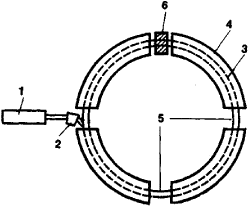Figure 6. Schematic of a weak-focusing electron or proton synchrotron: (1) injector, (2) injection system, (3) vacuum chamber, (4) electromagnet sector, (5) straight section, (6) accelerating device. The magnetic field is perpendicular to the plane of the figure.

The circular sections of the chamber are located in the gaps between the poles of the electromagnets that generate in the chamber the magnetic field needed to guide the motion of the particles in a closed orbit—that is, to bend the particle beam along the orbit. Since the radius of the equilibrium orbit must remain constant, the magnetic field must increase during acceleration from a value corresponding to the injection energy to a maximum value corresponding to the final energy. The increase in the magnetic field is accomplished by increasing the current flowing through the windings of the electromagnets. The shape of the magnet poles is selected so as to provide a small radial falloff of the magnetic field in accordance with condition (4). This falloff is necessary for stable motion of the particles in the transverse direction.

Accelerating devices that generate an alternating electric field are located in one or more straight sections. The field frequency varies strictly in accordance with the variation of the magnetic field [equation (7)]. The required precision of frequency reproduction is extremely great and is usually accomplished by means of an automatic frequency monitoring system using data on particle position. Since an error in frequency leads to departure of the particles from the equilibrium position, sensitive pickups are employed to register this departure, and their signal is amplified and used to make the required corrections in the frequency.

Under the action of the accelerating field the particles in the injected beam break up into bunches that group around stable equilibrium phases. The number of such bunches, which are located along the periphery of the accelerator, is equal to q. During acceleration the bunches become shorter in length by contracting toward the equilibrium phase. At the same time, a decrease occurs in the transverse dimensions of the beam, which at the start of acceleration occupies nearly the entire cross section of the vacuum chamber.

Alternating-gradient proton synchrotron. The alternating-gradient, or strong-focusing, proton synchrotron is distinguished above all by the arrangement of the magnet system, which consists of a large number of magnets in which a strong decrease and a strong increase in the magnetic field along the radius alternate. Particle focusing is much stronger in this case than in a weak-focusing accelerator. In one approach each magnet (Figure 7) performs the two functions of bending the particle beam in its orbit and focusing the beam. A separated-function design is also used. Magnets with uniform fields are used for bending, and focusing is accomplished by means of magnetic quadrupole lenses located in sections between the bending magnets.

The adoption of alternating-gradient magnetic systems entails more severe tolerances for magnet construction and mounting. For a ring magnet with a length greater than 1 km, the accuracy of alignment is measured in tenths or hundredths of a millimeter because of the high sensitivity of particle behavior to various random deviations of the magnetic field. In an inaccurately shaped field this sensitivity can lead to resonant buildup of particle oscillations.

Another characteristic of the alternating-gradient accelerator is the existence of a critical, or transition, energy. At a particle energy less than the critical energy, the stable equilibrium phase is located on the rising side of the voltage curve (phase – φ0 in Figure 1), since the period decreases with increasing energy, as in a linear accelerator. At a particle energy greater than the critical energy, by contrast, an increase in energy leads to an increase in the period revolution, as in a weak-focusing accelerator, and the phase + φ0 becomes the equilibrium phase. In order to prevent beam losses when the critical energy is passed, at the time of transition through the critical energy a rapid 2φ0 shift in the phase of the oscillations is introduced into the system, so that the particles being accelerated, which below the critical energy were grouped near the stable phase – φ0, end up in the region of the new stable phase + φ0.

A beam accelerated in a proton synchrotron may be fed to an internal target within the vacuum chamber. Alternatively, the beam may be extracted from the accelerator by means of a deflector that is of the same type used in the injection system but is more powerful because of the high particle speed. A new accelerating cycle then commences. The accelerating cycle repetition frequency in proton synchrotrons now in use is 5–30 cycles per minute. In each cycle 1011–1013 particles are accelerated. In principle the maximum intensity is determined by the limiting influence of the space charge.

Since a proton synchrotron capable of accelerating particles to very high energies requires very large dimensions and very severe tolerances in the construction of the machine (particularly the magnets), the feasibility of using superconducting materials in accelerator electromagnets and of employing methods of automatically controlling accelerator parameters is being explored. By using superconducting materials, magnetic fields at least three or four times greater than conventional fields can be obtained, and the size of the machine can be reduced by the same factor. By applying automatic control methods to accelerator parameters, the construction tolerances can be eased.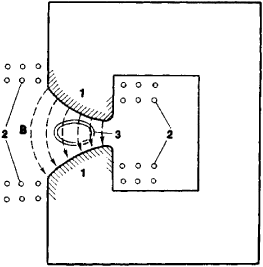Figure 7. Schematic cross section of a magnet of an alternating-gradient accelerator: (1) pole pieces shaped so as to provide a sharp change in the magnetic field along the radius, (2) electromagnet windings, (3) cross section of vacuum chamber, (B) magnetic field

ELECTRON SYNCHROTRON. The electron synchrotron is a circular resonance accelerator of electrons. The difference between the electron synchrotron and the proton synchrotron is that in the electron synchrotron only the magnetic field varies with time; the frequency of the accelerating electric field remains constant. At a constant frequency of revolution the orbit radius is proportional to the particle speed (R = v/ω); for electrons the speed becomes close to the speed of light at energies of the order of 1 MeV—that is, the speed changes little with increasing energy. Consequently, the radius of the equilibrium orbit in an electron synchrotron changes almost not at all. The magnet of the electron synchrotron, like that of the proton synchrotron, therefore has the form of a ring. Both weak- and strong-focusing electron synchrotrons are extremely similar to the proton synchrotron in design.

The maximum energies attainable in an electron synchrotron are determined primarily by the electromagnetic radiation of relativistic electrons. Since electrons moving in circular trajectories undergo centripetal acceleration, the laws of electrodynamics require that the electrons emit electromagnetic waves (seeSYNCHROTRON RADIATION). The energy emitted by an electron in one revolution is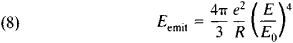where Ey = m0c2 is the rest energy of the particle, which for the electron is equal to 0.5 MeV. The energy emitted thus increases very rapidly with increasing electron energy. In large electron accelerators the energy emitted per revolution is comparable to the energy acquired by the particle. It may be noted that, in principle, electromagnetic radiation is generated when any charged particles move in a circle. For heavy particles (protons or nuclei), however, E0 is much greater than it is for electrons; consequently, heavy particles do not exhibit significant radiation at the energies achieved in accelerators.

The energy eV0 cos φ0 imparted to an electron by the accelerating field goes in part to increasing the particle energy and in part to radiation. The radiation affects particle oscillations about the equilibrium orbit. On the one hand, the radiation acts like friction in that it damps the oscillations of the particle. On the other hand, because the radiation is quantized and involves the emission of photons, the slowing down occurs not smoothly but in “clicks,” a circumstance that introduces additional buildup of oscillations.

Because of the large losses to radiation, the accelerating system must be of high power. Although the constant frequency of revolution makes possible the use of fixed-frequency resonant systems, the difficulty of building the accelerating system restricts the maximum attainable energies. By 1976 maximum energies of the order of 5–10 GeV had been reached in electron synchrotrons (see Table 3). Plans exist for electron synchrotrons capable of 100–150 GeV. In electron synchrotrons for lower energies (hundreds of millions of electron volts), betatron injection is often used instead of injection from an external preaccelerator, as in a proton synchrotron. When betatron injection is used, the accelerator initially operates as a betatron; after the electron reaches relativistic speeds (V ≈ c), the accelerating radio-frequency field is switched on, and the accelerator begins synchrotron operation.

SYNCHROCYCLOTRON. The synchrocyclotron, also known as the frequency-modulated cyclotron, is the third main type of circular resonance accelerator making use of the phase stability principle. In the synchrocyclotron the magnetic field is constant, and the frequency of the accelerating electric field varies; equation (3’) indicates that an increase in equilibrium energy requires a decrease in frequency. The synchrocyclotron is used to accelerate heavy particles (protons, deuterons, and alpha particles). The largest synchrocyclotrons now in use produce protons with a kinetic energy of up to 1,000 MeV.

In the synchrocyclotron particles move in spiral trajectories from the center, where a gas-discharge ion source is located, to the periphery of the vacuum chamber (Figure 8). They acquire energy through repeated passage across the accelerating gap. The accelerated particles either are used inside the vacuum chamber or are extracted to the outside by means of deflection systems. The accelerating-field frequency is changed by means of a variable capacitor in a resonant circuit. Because the particle orbit in a synchrocyclotron has a spiral shape, the synchrocyclotron requires a solid-core rather than ring magnet. As a result, the magnetic system is extremely bulky. Preference is therefore given to the proton synchrotron at energies above 1 GeV, although it produces accelerated beams of much lower intensity.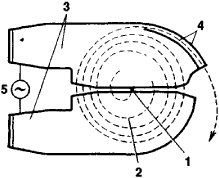Figure 8. Schematic of particle motion in the cyclotron and synchrocyclotron: (1) ion source, (2) spiral orbit of a particle undergoing acceleration, (3) accelerating electrodes, (4) extraction device (deflector plates), (5) source of accelerating field. The magnetic field is directed perpendicularly to the plane of the drawing.

In synchrocyclotrons with an azimuthally uniform magnetic field, vertical focusing is very weak, since n ≪ 1. For better vertical focusing, additional, azimuthal variations of the magnetic field are sometimes used—that is, alternating-gradient focusing is employed.

The three types of resonance accelerators described above, which make use of the phase stability principle, are characterized by pulsed operation: the group of particles bunched around the equilibrium phase increases its energy as the appropriate change

Table 3. Largest circular accelerators
Type and locationMaximum energy (GeV)Diameter of machine (m)Size of vacuum chamber (cm)Type of injectorEnergy at injection (MeV)Date of first operation
1As of 1976
Proton synchrotron
Dubna, USSR ...............107235 × 120Linac9.41957
Argonne, USA ...............12.75515 × 82Linac501963
Geneva, Switzerland ...............282007 × 15Linac501959
Booster8001972
Brookhaven, USA ...............332578 × 1 7Linac2001960
Serpukhov, USSR ...............7647212 × 20Linac1001967
Booster
Batavia, USA ...............50012,0005 × 13Booster8,0001972
Electron synchrotron
Daresbury, Great Britain ...............5.270(4–6) × (11–15)Linac431966
Yerevan, USSR ...............6.1693 × 1 0Linac501967
Hamburg, Federal Republic of Germany ...............7.5101(4–7) × (10–12)Linac300–5001964
Cornell University, Ithaca, USA ...............12.22502.5 × 5.5Linac1501967
Synchrocyclotron
Geneva, Switzerland ...............0.605.01957
Dubna, USSR ...............0.686.01953

in the accelerating-field frequency and/or magnetic induction occurs. After maximum energy is reached, the group of particles either is used inside the vacuum chamber or is extracted from the accelerator. The accelerator parameters then revert to their initial values, and a new accelerating cycle begins. The duration of the acceleration pulse is of the order of hundredths of a second in electron synchrotrons and synchrocyclotrons and of the order of a few seconds in proton synchrotrons.

CYCLOTRON. The cyclotron is a circular resonance accelerator for protons or ions. In this machine both the magnetic field and the frequency of the accelerating electric field are constant. Unlike accelerators described previously, the cyclotron provides continuous output. It is similar in design to the synchrocyclotron.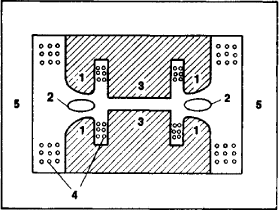Figure 9. Schematic cross section of a betatron: (1) magnet poles, (2) cross section of annular vacuum chamber, (3) central core, (4) electromagnet windings, (5) magnet yoke

In a cyclotron particles from the ion source continuously enter the vacuum chamber, where they are accelerated by electrodes and move in a spiral. The quantities ωa and B do not vary with time in a constant-gradient cyclotron, but the particle energy increases. Consequently, resonance condition (3’) is violated, and resonance acceleration can occur only as long as the kinetic energy W acquired is much less than the rest energy m0c2—that is, only until the relativistic increase in the particle’s mass begins to manifest itself. This fact determines the upper limit of the energies attainable in a cyclotron; for protons the limit is approximately 10–20 MeV. The limiting energy is achieved at very high voltages on the accelerating electrodes. On the other hand, because of its continuous operation, the cyclotron provides a high beam intensity. The magnetic field in a cyclotron has a very small radial falloff; a large falloff of the field, it may be noted, would intensify still further the deviation from exact resonance. Magnetic-field focusing in the vertical direction is therefore very weak (n ≈ 0), especially at the center of the magnet. In the central region, however, the particle speeds are still small, and electric-field focusing has a substantial influence.

Exact resonance between a particle and the constant-frequency accelerating field could be maintained in a cyclotron if the magnetic field increased with radius. In a constant-gradient accelerator such an increase is impermissible because of the resulting instability of motion in the vertical direction. If, however, alternating-gradient focusing is used, stable acceleration can be achieved to much higher energies than in ordinary cyclotrons. Machines of this type are called isochronous, or sector, cyclotrons. They have the same advantage of high intensity as cyclotrons have and are capable of producing intense proton beams at energies of up to 1,000 MeV. The isochronous cyclotron at the Swiss Institute for Nuclear Research delivers a proton current of 12 microamperes (µ A); the maximum energy of the accelerated particles in the cyclotron is 590 MeV.

MICROTRON. The microtron, or electron cyclotron, is a circular resonance accelerator in which, as in the cyclotron, both the magnetic field and the accelerating-field frequency are constant in time. In a microtron, however, resonance is maintained during acceleration through a variation in q.

A particle revolves in a microtron in a uniform magnetic field and repeatedly passes through an accelerating cavity. The particle’s energy gain in the cavity is such that the particle’s period of revolution changes by an amount that is equal to or a multiple of the accelerating-voltage period. If the particle revolves in resonance with the accelerating field from the very start, this resonance is maintained despite the change in the period of revolution. Suppose, for example, the particle makes its first revolution in one period of the accelerating field (that is, q = 1), its second revolution in two periods (q = 2), its third revolution in three periods (q = 3), and so on. Clearly, the particle always crosses the accelerating cavity at the same phase. A type of phase stability exists in the microtron, so that near-equilibrium particles are also accelerated.

The microtron produces a continuous output. Some machines are capable of delivering currents of the order of 100 milliamperes (mA). The maximum energy reached is of the order of 30 MeV, which has been attained in machines in the USSR and Great Britain. It is difficult to reach higher energies because of the severe magnetic-field tolerances required; substantial increases in current are limited by the synchrotron radiation of the electrons being accelerated.

For prolonged maintenance of resonance, the magnetic field of a microtron must be uniform. Since such a field does not have vertical focusing properties, the focusing is accomplished with the electric field of the accelerating cavity. Modifications of the microtron have been proposed with an azimuthally varying magnetic field (the sector microtron), but they have not undergone serious development.

BETATRON. The betatron, which is used to accelerate electrons, is the only nonresonance-type circular accelerator.

Electrons are accelerated in the betatron by means of the vorticity electric field induced by time-varying magnetic flux passing through the core, or central part, of a magnet. An annular vacuum chamber is located in the gap between the magnet poles. The pole faces are specially shaped so as to impart a small radial falloff to the magnetic field; the field causes revolution of the particles in a circle and provides focusing of the particles with respect to the average equilibrium radius (Figure 9). In order for the orbit radius to remain constant, there must exist a definite relation, called the betatron condition, between the rate of energy increase, which is determined by the change in the field in the central part, and the rate of increase of the magnetic guide field. The relation reduces to the condition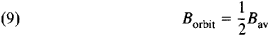which means that the field at the orbit (Borbit) should be half the average field (Bav) within the orbit. When this condition and focusing condition (4) are satisfied, stable acceleration of particles occurs in an orbit of constant radius.

The betatron has a pulsed output and can be used as a source of electrons of energies of the order of 100–300 MeV. For energies above 100–200 MeV, however, the electron synchrotron, which does not have a bulky central core, is more convenient. Betatrons for energies of 20–50 MeV are particularly common; they are used for various purposes and are lot produced. As noted above, betatron-type acceleration is often used in electron synchrotrons for preacceleration. Since such acceleration is to a low energy, the core required for betatron operation is small and does not substantially complicate the design of the synchrotron.

Linear accelerators, ELECTROSTATIC ACCELERATOR. Electrostatic accelerators are discussed in ACCELERATOR, HIGH-VOLTAGE.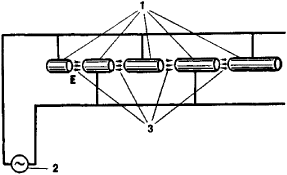Figure 10. Schematic of a Wideröe-type linear accelerator: (1) drift tubes, (2) source of alternating voltage, (3) region of action of electric field E

LINEAR INDUCTION ACCELERATOR. In a linear induction accelerator particles are accelerated by the electromotive force induced upon a change in a ring-shaped magnetic field. Ferromagnetic rings surrounded by current windings are mounted along the accelerator axis. A sharp change in the current in the windings causes a rapid change in the magnetic field. In accordance with the law of electromagnetic induction, this change in the magnetic field generates an electric field E at the accelerator axis. A charged particle traveling along the axis during the existence of this field acquires an energy eEL, where L is the distance traversed. For the accelerating field to be sufficiently great, the magnetic field must be changed rapidly. The lifetime of the accelerating field and, consequently, the duration of the acceleration pulse are therefore small (of the order of 10–9–10–6 sec).

The advantages of linear induction accelerators are large accelerated-particle currents (hundreds or thousands of amperes), high beam uniformity (a small energy spread and small velocities of transverse motion), and high efficiency of conversion of power consumed by the accelerating system into output beam power. Existing linear induction accelerators produce electron beams having an energy of a few million electron volts and are used principally as sources of intense relativistic-electron beams in collective acceleration machines and in devices for research on thermonuclear fusion. The linear induction accelerator has, however, potentially much broader application.

LINEAR RESONANCE ACCELERATORS. Linear resonance machines constitute the most widely used type of linear accelerator, especially at high energies. Linear resonance accelerators for electrons produce energies ranging from tens of millions of electron volts to ~20 GeV; machines for protons produce energies of up to 800 MeV. The substantial difference in energy between proton and electron linear accelerators is due chiefly to the circumstance that protons are accelerated to nonrelativistic or slightly relativistic speeds, whereas electrons can be accelerated to very high relativistic speeds. Proton linear accelerators designed for energies of ~600–800 MeV, at which relativistic effects become appreciable, approach electron linear accelerators in design. The parameters of the largest linear accelerators are given in Table 4.

Proton linear resonance accelerator. The idea of the linear resonance accelerator was advanced in 1924 by the Swedish scientist G. Ising and was implemented by Wideröe in 1928. This type of accelerator (Figure 10) consists of a system of hollow cylinders, called drift tubes, connected alternately to opposite poles of an alternating-voltage source. The electric field does not penetrate the tubes and is concentrated in the gaps between them. The lengths of the tubes are such that if particles enter the first gap between tubes at a time when the field is directed so as to accelerate the particles, they will also enter subsequent gaps at an accelerating phase of the field. Thus, the particles’ energy will continually increase, and we may speak of resonance acceleration. An accelerator of approximately this type was built in 1931 by Lawrence and D. Sloan of the USA.

Advances in high-frequency radio engineering in the 1940’s gave further impetus to the development of proton linear accelerators. Instead of lumped-constant circuits, modern proton accelerators generally use the scheme proposed by the American physicist L. Alvarez, which involves a resonant cavity with drift tubes. Within the cavity, which is of cylindrical shape, an alternating electric field directed along the cavity’s axis is generated. The particles being accelerated pass through the system of drift tubes in such a way that they cross the accelerating gaps between tubes at times when the field is directed with the particle motion (Figure 11). When the field is directed in the opposite direction, the particles are located inside the tubes, which the field cannot penetrate.

A linear resonance accelerator, as indicated above, makes use of phase stability, so that the particles located in some region about the equilibrium particle are accelerated together with it and, on the average, acquire the same energy. The stable equilibrium phase in a linear accelerator is negative—that is, is located in the interval where the field rises. The electric field therefore has a defocusing action in a linear accelerator, and special steps must be taken to provide focusing of the protons. In low-energy accelerators foil or grid focusing may be employed: the input apertures of the drift tubes are covered with a foil or grid barrier. This approach deforms the field between tubes, and the defocusing region vanishes almost entirely. In high-energy accelerators this focusing method cannot be used, since the foils and grids cause intolerable losses of intensity; moreover, the foils and grids burn up under the action of the beam. The most widely used focusing method is alternating-gradient focusing with magnetic quadrupole lenses located inside the drift tubes. The lenses produce in the vicinity of the accelerator axis a magnetic field that increases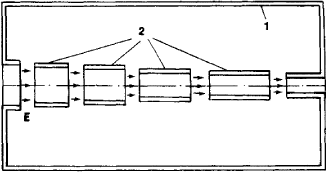Figure 11. Schematic cross section of the Alvarez linear accelerator structure: (1) cavity, (2) drift tubes. Near the axis the electric field E is found only in the gaps between tubes.

linearly with distance from the axis. Qualitatively, focusing by means of such a field can be explained in the same way as for circular accelerators.

The advantages of linear accelerators over circular accelerators include the absence of a cumbersome magnetic system, simplicity of particle injection and extraction, and high current densities. The capabilities of proton linear accelerators are limited, however, by the complexity and high cost of the radio-engineering system required and by the difficulty of focusing. At the present time proton linear accelerators are used primarily as injectors for ring accelerators. The injectors reach an energy of 50–100 MeV or even 200 MeV. At higher energies the Alvarez structure becomes inefficient from a radio-engineering standpoint, since too much power is required in order to produce the electric field (the shunt impedance is too low). Special systems of coupled cavities have been developed for acceleration to higher energies. A waveguide system with diaphragms may also be used, as in electron linear accelerators.

Table 4. Largest linear accelerators
Type and locationDate of first operationMaximum energy (MeV)Length (m)Duration of pulse of accelerated particles (μ sec)Maximum average current (μA)Maximum current in pulse (mA)
Electron linear accelerator Kharkov, USSR ...............19641,8002401.40.8
Stanford University, Palo Alto, USA ...............196622,3003,0501.648
Proton linear accelerator
Serpukhov, USSR (injector) ...............196710080300 180
Batavia, USA (injector) ...............1970200145400 120
Los Alamos, USA (meson factory) ...............19728007955001,000
Academy of Sciences, USSR (meson factory) ...............Under construction600450100500

Present-day high-energy linear accelerators of protons consist of two stages: in the first stage, acceleration is carried out to 100–200 MeV with Alvarez-type cavities; the second stage has cavities of different type whose characteristics are more favorable at such particle energies. This two-stage scheme is used in the 800-MeV proton linear accelerator at Los Alamos in the USA, which can produce an average current of 1,000 µA. The facility is used for physics experiments with intense secondary beams and is sometimes referred to as a meson factory. A 600-MeV meson factory using the same scheme is under construction in the USSR.

Electron linear resonance accelerator. Electron linear resonance accelerators have an additional advantage over circular accelerators: because the velocity of the electrons is practically constant in both magnitude and direction, electrons in linear accelerators emit almost no radiation. The maximum energy reached by present-day electron linear accelerators is ~20 GeV, but this upper limit is dictated solely by economic considerations and could be raised by simply increasing the length.

For electron linear accelerators, in which particles travel almost from the very beginning at a speed close to that of light, the most advantageous accelerating system is a traveling-wave structure in the form of a diaphragm-loaded wave guide. In a wave guide with smooth walls, electromagnetic waves travel with a phase speed that exceeds the speed of light. In order for a traveling wave to be able to accelerate particles, it must move with the same speed as the particles—that is, to accelerate electrons, the wave must be slowed down to the speed of light. This slowing down can be accomplished by, for example, introducing diaphragms into the wave guide (Figure 12).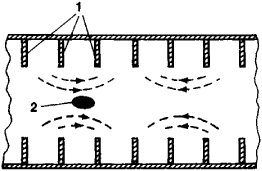Figure 12. Schematic cross section of a diaphragm-loaded wave guide: (1) diaphragms, (2) electron bunch undergoing acceleration. The arrows indicate the configuration of the field traveling along the wave guide.

Since the electron speed is close to the speed of light, the motion of the electrons with respect to the accelerating waves exhibits special characteristics. For example, the phase stability principle does not apply to the electrons: a change in the energy of an electron causes almost no change in its speed and, consequently, does not lead to a shift relative to the accelerating wave. Focusing in the transverse direction is also generally unnecessary, because the speeds of the electrons’ random transverse motions decrease as the electrons’ energy increases. (The law of conservation of momentum requires that the transverse momentum mv remain constant; moreover, according to the theory of relativity, the mass m increases with increasing energy. Consequently, the transverse speed v decreases.) Furthermore, transverse Coulomb repulsion in electron accelerators is almost canceled by the magnetic attraction of parallel currents. The electron bunches being accelerated can, however, excite in the accelerating wave guide spurious waves that lead to beam blowup. This effect is of particular importance in large accelerators, where it limits the maximum attainable currents. A number of engineering methods of suppressing the effect have been developed.

Electron linear accelerators operating at low energies of the order of tens of millions of electron volts are widely encountered. The accelerators are used for research in nuclear and neutron physics and have many practical applications.

Intensive studies are being carried out on the feasibility of using superconducting materials for the walls of cavities and wave guides in proton and electron linear accelerators. Such materials would greatly reduce the consumption of microwave power and would make possible continuous, rather than pulsed, operation of linear accelerators.

Accelerators for particles other than electrons and protons. The type of particle accelerators described above can be used to accelerate not only electrons and protons but also other charged particles. With practically no modifications electron accelerators can be employed to accelerate positrons. Various types of proton accelerators are used to accelerate heavy particles. The highest ion energy has been achieved in the Bevalac accelerator in the USA. This machine, which is based on a proton synchrotron, in 1974 accelerated nuclei as heavy as the argon nucleus to an energy of 2 GeV per nucleon. At Dubna plans have been drawn up for an accelerator, called a nuklotron, that would be capable of reaching 16 GeV per nucleon. Linear accelerators and cyclotron-type accelerators are also used as sources of heavy ions.

REFERENCES

Grinberg, A. P. Metody uskoreniia zariazhennykh chastits. Moscow-Leningrad, 1950.
Uskoriteli (collection of articles). Moscow, 1962. (Translated from English and German.)
Kolomenskii, A. A. and A. N. Lebedev. Teoriia tsiklicheskikh uskoritelei. Moscow, 1962.
Bruck, H. Tsiklicheskie uskoriteli zariazhennykh chastits. Moscow, 1970. (Translated from French.)
Val’dner, O. A., A. D. Vlasov, and A. V. Shal’nov. Lineinye uskoriteli. Moscow, 1969.
Komar, E. G. Osnovy uskoritel’noi tekhniki. Moscow, 1975.
Sokolov, A. A., and I. M. Ternov. Reliativistskii elektron. Moscow, 1974.

E. L. BURSHTEIN

particle accelerator

[′pärd·ə·kəl ik′sel·ə‚rād·ər]
(nucleonics)
A device which accelerates electrically charged atomic or subatomic particles, such as electrons, protons, or ions, to high energies. Also known as accelerator; atom smasher.

particle accelerator

An electrical device that generates charged particles, such as electrons, protons and ions, at high energy. So-called "nuclear accelerators" are used to split the atom for scientific research, but most particle accelerators are built for more practical applications. They are used to manufacture myriad products including semiconductors. They are also used as X-ray machines for cancer treatment and for detecting weaknesses in materials.

Splitting Atoms
The Large Hadron Collider (LHC) at CERN, the European laboratory for nuclear research, is the world's largest particle accelerator. Online in 2008 and costing around USD \$6 billion, protons are made to collide into other particles 10 million times per second while making 17-mile laps at nearly the speed of light. One of the main goals of the LHC was to find the Higgs boson, called the "God Particle," considered to be the fundamental element of matter. See Higgs boson.

In order to process the data coming from the accelerator, a huge computing grid uses resources from more than 150 institutions around the world. See peer-to-peer computing.

Particle Accelerator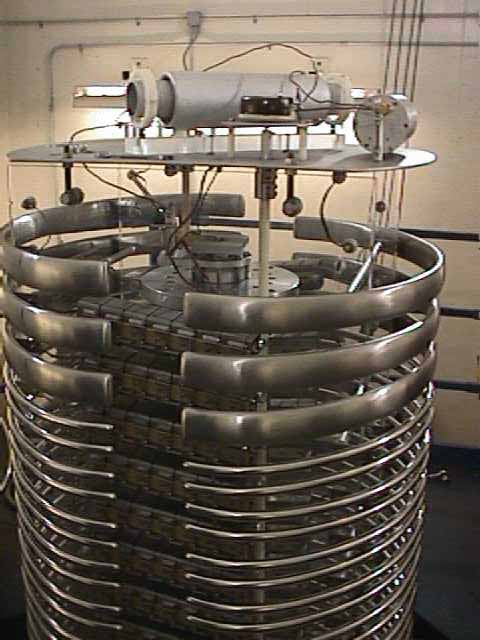Looking like science fiction, this accelerator is used for a very mundane application. Standing about 10 feet tall, it radiates plastic heat shrinkable tubing to give it a memory. The tubing is then moved to a machine that increases its diameter. Used to protect wires and connections in electronic and electrical circuits, when the tubing is placed over the wires and heated, it returns to its original size because of the radiation.
Site: Follow: Share:
Open / Close